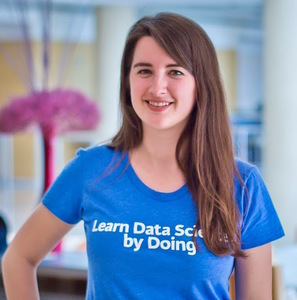# Time Series Analysis in R

Learn the core techniques necessary to extract meaningful insights from time series data.
4 Hours16 Videos58 Exercises44,670 Learners
4600 XP

or
By continuing you accept the Terms of Use and Privacy Policy. You also accept that you are aware that your data will be stored outside of the EU and that you are above the age of 16.

## Course Description

Many phenomena in our day-to-day lives, such as the movement of stock prices, are measured in intervals over a period of time. Time series analysis methods are extremely useful for analyzing these special data types. In this course, you will be introduced to some core time series analysis concepts and techniques.

1. 1

### Exploratory time series data analysis

Free
This chapter will give you insights on how to organize and visualize time series data in R. You will learn several simplifying assumptions that are widely used in time series analysis, and common characteristics of financial time series.
2. 2

### Predicting the future

In this chapter, you will conduct some trend spotting, and learn the white noise (WN) model, the random walk (RW) model, and the definition of stationary processes.
3. 3

### Correlation analysis and the autocorrelation function

In this chapter, you will review the correlation coefficient, use it to compare two time series, and also apply it to compare a time series with its past, as an autocorrelation. You will discover the autocorrelation function (ACF) and practice estimating and visualizing autocorrelations for time series data.
4. 4

### Autoregression

In this chapter, you will learn the autoregressive (AR) model and several of its basic properties. You will also practice simulating and estimating the AR model in R, and compare the AR model with the random walk (RW) model.
5. 5

### A simple moving average

In this chapter, you will learn the simple moving average (MA) model and several of its basic properties. You will also practice simulating and estimating the MA model in R, and compare the MA model with the autoregressive (AR) model.
In the following tracks
Quantitative AnalystTime Series
CollaboratorsLore DirickMatt Isaacs
Prerequisites
Intermediate R#### David S. Matteson

Associate Professor at Cornell University
David S. Matteson is Professor of Statistical Science at Cornell University and co-author of Statistics and Data Analysis for Financial Engineering with R examples.

## What do other learners have to say?

I've used other sites—Coursera, Udacity, things like that—but DataCamp's been the one that I've stuck with.

Devon Edwards Joseph
Lloyds Banking Group

DataCamp is the top resource I recommend for learning data science.

Louis Maiden